# Performance Monitoring with EPOS

## Definition

This page describes an example of how to collect performance data in execution time using EPOS.
The process used was based on the use of PMU and other MSR’s statistics and it focused on I/O and memory hierarchy aspects on a Real-time system on a Multi-core IA32 architecture.

## Scheduler Criteria Development

The performance monitoring, even executing on strategically chosen areas, causes jitter on execution process, so, to permit user to have control on it’s execution we created a new scheduler criteria.
Only using this as the chosen Criterion on traits file will cause to initialize the monitoring algorithm.

### Init method

One of the changes made to give user the option to choose between use or not the performance monitoring, was to add an empty method on the Priority Class, so we can configure the PMU and any other monitoring useful thing with an override of this method into the developed Scheduler Criteria.

```class Priority
{

public:
enum {
MAIN   = 0,
HIGH   = 1,
NORMAL = (unsigned(1) << (sizeof(int) * 8 - 1)) - 3,
LOW    = (unsigned(1) << (sizeof(int) * 8 - 1)) - 2,
IDLE   = (unsigned(1) << (sizeof(int) * 8 - 1)) - 1
};

static const bool timed = false;
static const bool dynamic = false;
static const bool preemptive = true;
static const bool energy_aware = false;

public:
Priority(int p = NORMAL): _priority(p) {}

operator const volatile int() const volatile { return _priority; }

static void init() {} //ADDED METHOD

void update() {}
unsigned int queue() const { return 0; }

protected:
volatile int _priority;
};```

### MCEDF Criterion

This is the new Scheduler Criteria, it extends the CEDF Scheduler. (MCEDF - Monitored CEDF)

```class MCEDF : public CEDF
{
enum { ANY = Variable_Queue::ANY };
public:
static const bool energy_aware = true;
public:
static void init() {
PMU::stop(0);
PMU::stop(1);
PMU::stop(2);
PMU::stop(3);
PMU::stop(4);
PMU::stop(5);
PMU::stop(6);

PMU::reset(0);
PMU::reset(1);
PMU::reset(2);
PMU::reset(3);
PMU::reset(4);
PMU::reset(5);
PMU::reset(6);

PMU::write(0, 0);
PMU::write(1, 0);
PMU::write(2, 0);
PMU::write(3, 0);
PMU::write(4, 0);
PMU::write(5, 0);
PMU::write(6, 0);

PMU::config(3, PMU::INSTRUCTION);
PMU::config(4, PMU::L2_LINES_IN_ALL);
PMU::config(5, PMU::L1D_REPLACEMENT);
PMU::config(6, PMU::L2_TRANS_L1D_WB);

PMU::start(0);
PMU::start(1);
PMU::start(2);
PMU::start(3);
PMU::start(4);
PMU::start(5);
PMU::start(6);
}
MCEDF(int p = APERIODIC)
: CEDF(p) {} // Aperiodic

MCEDF(const Microsecond & d, const Microsecond & p = SAME, const Microsecond & c = UNKNOWN, int cpu = ANY)
: CEDF(d, p, c, cpu) {}

using Variable_Queue::queue;

static unsigned int current_queue() { return Machine::cpu_id() / HEADS; }
};```

As we can see on the code above, the initialization and configuration of PMU is done inside the method init.
On the next topics we will describe how the System initialization pass through it (call this method).

## PMU Initialization (Configuration) - Problem Description

The first solution used to call the init method of the Scheduler Criteria was to put a simple call into “Thread::init” where all the CPUS are supposed to pass on the System initialization.
But after try it, we observer that only CPU 0 executed the “Thread::init” code. It was possible to be observed looking at the tests described also at this project https://epos.lisha.ufsc.br/Adaptive+DVFS+for+EPOS+Multicore+Schedulers
where after running the tests using the PMU architectural event INSTRUCTION_RETIRED.
Printing the contabilized value we see 0’s on every CPU except the CPU 0, what is not the expected value after the test execution.

```void Thread::init()
{
// The installation of the scheduler timer handler must precede the
// creation of threads, since the constructor can induce a reschedule
// and this in turn can call timer->reset()
// Letting reschedule() happen during thread creation is harmless, since
// MAIN is created first and dispatch won't replace it nor by itself
// neither by IDLE (which has a lower priority)
if(Criterion::timed && (Machine::cpu_id() == 0))
_timer = new (SYSTEM) Scheduler_Timer(QUANTUM, time_slicer);

// Install an interrupt handler to receive forced reschedules
if(smp) {
if(Machine::cpu_id() == 0)
IC::int_vector(IC::INT_RESCHEDULER, rescheduler);
IC::enable(IC::INT_RESCHEDULER);
}

//cout << "CPU:" <<Machine::cpu_id()<< " passou aqui"<< endl; -> this was used to print which CPU executes the code
//_cpu_temperature[Machine::cpu_id()] = Thermal::temperature();

//Inicialization of PMU Channels to the statistics collecting in energy aware policies
// Capturing INSTRUCTION, LLC_MISS, DVS_CLOCK.
if(Criterion::energy_aware) {
_cpu_temperature[Machine::cpu_id()] = Thermal::temperature(); // first thermal read
}

Criterion::init(); //it is executed for every Criterion, but only one of them is not empty
}```

### Test showing the error

```// EPOS Thread Component Initialization

#include <system.h>
#include <alarm.h>
#include <utility/ostream.h>

__BEGIN_SYS

OStream cout;

{
// The installation of the scheduler timer handler must precede the
// creation of threads, since the constructor can induce a reschedule
// and this in turn can call timer->reset()
// Letting reschedule() happen during thread creation is harmless, since
// MAIN is created first and dispatch won't replace it nor by itself
// neither by IDLE (which has a lower priority)
if(Criterion::timed && (Machine::cpu_id() == 0))
_timer = new (SYSTEM) Scheduler_Timer(QUANTUM, time_slicer);

// Install an interrupt handler to receive forced reschedules
if(smp) {
if(Machine::cpu_id() == 0)
IC::int_vector(IC::INT_RESCHEDULER, rescheduler);
IC::enable(IC::INT_RESCHEDULER);
}

cout << "CPU:" <<Machine::cpu_id()<< " passou aqui"<< endl;
//_cpu_temperature[Machine::cpu_id()] = Thermal::temperature();

//Inicialization of PMU Channels to the statistics collecting in energy aware policies
// Capturing INSTRUCTION, LLC_MISS, DVS_CLOCK.
if(Criterion::energy_aware) {
_cpu_temperature[Machine::cpu_id()] = Thermal::temperature();

for (unsigned int i = 0; i < Machine::n_cpus(); i++) {
_cpu_monitor[(int)i] = new Monitoring_Capture();
}
}

Criterion::init();

}

__END_SYS```

#### Code on “Criterion::init()” - PMU CONFIG

```class MCEDF : public CEDF
{
enum { ANY = Variable_Queue::ANY };
public:
static const bool energy_aware = true;
public:
static void init() {
PMU::stop(0);
PMU::stop(1);
PMU::stop(2);
PMU::stop(3);
PMU::stop(4);
PMU::stop(5);
PMU::stop(6);

PMU::reset(0);
PMU::reset(1);
PMU::reset(2);
PMU::reset(3);
PMU::reset(4);
PMU::reset(5);
PMU::reset(6);

PMU::write(0, 0);
PMU::write(1, 0);
PMU::write(2, 0);
PMU::write(3, 0);
PMU::write(4, 0);
PMU::write(5, 0);
PMU::write(6, 0);

PMU::config(3, PMU::INSTRUCTION); // we only printed this code
PMU::config(4, PMU::L2_LINES_IN_ALL);
PMU::config(5, PMU::L1D_REPLACEMENT);
PMU::config(6, PMU::L2_TRANS_L1D_WB);

PMU::start(0);
PMU::start(1);
PMU::start(2);
PMU::start(3);
PMU::start(4);
PMU::start(5);
PMU::start(6);
}
MCEDF(int p = APERIODIC)
: CEDF(p) {} // Aperiodic

MCEDF(const Microsecond & d, const Microsecond & p = SAME, const Microsecond & c = UNKNOWN, int cpu = ANY)
: CEDF(d, p, c, cpu) {}

using Variable_Queue::queue;

static unsigned int current_queue() { return Machine::cpu_id() / HEADS; }
};```

#### Test Application code

```cout << "\n\n\n\n\n Channel-3:" << endl;
for (int i = 0; i < Machine::n_cpus(); i++) {
cout << "\n\n\n\n\nEvt3" << i << endl;
for (int j = 0; j < Thread::_tempPos[i]; j++)
}
//prints only the channel 3 of the PMU read by all the THREADS```

#### On the following log the label EvtNM is Event number N of the CPU M

```<0>: PCI: device [0:2.0] reports implausible large region. Ignoring! :<0>
Setting up this machine as follows:
Processor:    8 x IA32 at 3392 MHz (BUS clock = 12 MHz)
Memory:       262144 Kbytes [0x00000000:0x10000000]
User memory:  261700 Kbytes [0x00000000:0x0ff91000]
PCI aperture: 5142 Kbytes [0xfe000000:0xfe505800]
Node Id:      will get from the network!
Setup:        22624 bytes
APP code:     33728 bytes	data: 1369312 bytes

CPU:0 passou aqui

Channel-3:

Evt30
106457
109726
110761
2242585
2242852
2243321

Evt31
0
0
0
0
0
0

Evt32
0
0
0
0
0
0

Evt33
0
0
0
0
0
0

Evt34
0
0
0
0
0
0

Evt35
0
0
0
0
0
0

Evt36
0
0
0
0
0
0

Evt37
0
0
0
0
0
0```

### Solution

To solve the problem on the System initialization, we decided to change the code into some classes as it follows on the next topics.

#### System Init

Here, we added a if structure to guarantee only CPU 0 will execute some actions. The code is bellow.

```// EPOS System Component Initialization

#include <system.h>
#include <alarm.h>

__BEGIN_SYS

void System::init()
{
if (Machine::cpu_id() == 0) {
if(Traits<MMU>::colorful)
Page_Coloring::init();

if(Traits<Alarm>::enabled)
Alarm::init();
}

}

__END_SYS```

#### init_system

In this file (init_system.cc) we added a code to guarantee the other CPU’s will access the System_init.

```// EPOS System Initializer

#include <utility/random.h>
#include <machine.h>
#include <system.h>
#include <segment.h>
#include <pmu.h>

__BEGIN_SYS

class Init_System
{
private:
static const unsigned int HEAP_SIZE = Traits<System>::HEAP_SIZE;

public:
Init_System() {
db<Init>(TRC) << "Init_System()" << endl;

Machine::smp_barrier();

// Only the boot CPU runs INIT_SYSTEM fully
if(Machine::cpu_id() != 0) {
// Wait until the boot CPU has initialized the machine
Machine::smp_barrier();
// For IA-32, timer is CPU-local. What about other SMPs?
Timer::init();

if(Traits<PMU>::enabled)
PMU::init();		//CPU0 calls PMU::init() inside CPU::init()

if(Traits<Thread>::enabled) //here is the code placed to allow the other cpus to access system init
System::init(); //the call

return;
}
...```

Here we call Criterion::init(), witch configure the PMU events if the Criterion is MCEDF, otherwise it will not do anything.

```#include <system.h>
#include <alarm.h>

__BEGIN_SYS

{
if(Criterion::timed && (Machine::cpu_id() == 0))
_timer = new (SYSTEM) Scheduler_Timer(QUANTUM, time_slicer);
if(smp) {
if(Machine::cpu_id() == 0)
IC::int_vector(IC::INT_RESCHEDULER, rescheduler);
IC::enable(IC::INT_RESCHEDULER);
}

if(Criterion::energy_aware) {
_cpu_temperature[Machine::cpu_id()] = Thermal::temperature();
}
//Inicialization of PMU Channels to the statistics collecting in energy aware policies
// only happens if the criterion is MCEDF
Criterion::init();
}
__END_SYS```

### Working Test

Now we will print all the CPU’s, so to avoid printing characters out of order, what can turn the reading into an almost impossible task, we will print only the number of the CPU.

```__BEGIN_SYS

OStream cout;

{
// The installation of the scheduler timer handler must precede the
// creation of threads, since the constructor can induce a reschedule
// and this in turn can call timer->reset()
// Letting reschedule() happen during thread creation is harmless, since
// MAIN is created first and dispatch won't replace it nor by itself
// neither by IDLE (which has a lower priority)
if(Criterion::timed && (Machine::cpu_id() == 0))
_timer = new (SYSTEM) Scheduler_Timer(QUANTUM, time_slicer);

// Install an interrupt handler to receive forced reschedules
if(smp) {
if(Machine::cpu_id() == 0)
IC::int_vector(IC::INT_RESCHEDULER, rescheduler);
IC::enable(IC::INT_RESCHEDULER);
}

//cout << "CPU:" <<Machine::cpu_id()<< " passou aqui"<< endl;
cout <<Machine::cpu_id()<<endl; //this line instead of the above
...```

#### Result

```<0>: PCI: device [0:2.0] reports implausible large region. Ignoring! :<0>
Setting up this machine as follows:
Processor:    8 x IA32 at 3392 MHz (BUS clock = 12 MHz)
Memory:       262144 Kbytes [0x00000000:0x10000000]
User memory:  261700 Kbytes [0x00000000:0x0ff91000]
PCI aperture: 5142 Kbytes [0xfe000000:0xfe505800]
Node Id:      will get from the network!
Setup:        22624 bytes
APP code:     33664 bytes	data: 1369312 bytes
01257
4
36

Channel-3:

Evt30
3519217
3521826
3522841
5653739
5654006
5654341
5654810

Evt31
6812288
6812652
6812887
6813286
6813521
6813975
8945272

Evt32
1730055
1730315
1731020
3862065
3862332
3862592
3862852

Evt33
1744255
1744527
1745054
1745289
1746243
3877077
3877344

Evt34
2965639
2965899
2966738
5097732
5097999
5098263
5098523

Evt35
8807
9067
9486
2140420
2140687
2140947
2141207

Evt36
1755373
1755633
1756052
3888386
3888653
3888913
3889173

Evt37
3357735
3357995
3358610
5489786
5490053
5490321
5490581```

As we can see on the result above, all the CPU’s printed values different of 0, also, in the first three (3) lines of the log it was printed the numbers from 0 to 7, what means that every CPU (0 - 7, or 8 CPU’s) executed the “Thread::init()” code.

## PMU Version

Defined on include/architecture/ia32/traits.h

```…
template<> struct Traits<PMU>: public Traits<void>
{
static const bool enabled = true;
enum { V1, V2, V3, DUO, MICRO, ATOM, SANDY_BRIDGE };
static const unsigned int VERSION = SANDY_BRIDGE;

static const bool pmc_interrupt_enabled = true;
static const unsigned int pmc_handler_period = 500000;
};
...```

So, as the server we used for tests uses Sandy Bridge microarchitecture, we needed to set it as Sandy Bridge to use it’s events.

### Sandy-Bridge Events

The Sandy-Bridge events are listed on the Intel Software Developer's Manual at chapter 19, more specifically on the table 19-13 at page 339 of the following link that contains only the volume 3b part 2 of the manual: Intel SDM - 3b

### Pre filtering

To execute some tests in order to be sure about the correctness of the configuration made and, also take a notion about which events shows impact with certain kind of execution.

To make this tests we used a sequence of memory copies made by every thread (it was one thread per logical CPU).

The tested events list can be find at Link to the events list (PDF) and the resultant logs of those tests are available at Link to test logs. The files description can be found at the first link of this topic (Pre filtering).

## Data Collecting

There were two things to be defined here. First where to collect and then the data collecting structure.
They are both described bellow.

### Where to Collect

• Idle: Depending on the load and scheduling, you can get in a lot here (generating an overhead of equal data). Depending on the load and scaling, you can never enter here or very seldom.

• Dispatch: Bigger entry guarantee (hardware interruption), but with the experience we had in our previous work {3}, it seems to come in a few times. But it may have been the load of our test.

• Idle and Dispatch: Good combination, previously used in ADVFS work {3}, but should be used with caution as it can generate overhead. (Chosen option)

### Data Collecting Structure

The first thing done here was to make a brainstorm of the possible data structures and so, analyze the positive and negative aspects of each one. This would make easier to take a decision.

#### Options

• Vector: Its usage depends on how much data must be stored. It is easy to implement but it's size must be fixed before the execution.
• An alternative to use vectors is to use circular vectors, this would cause each time the vector reached its limit, there be a flush (in this case, sending the data) and the data overwriting (delete the current element at the position to be written and then write the new element).

• Linked List: Its usage does not depend on the amount of data to be stored, but it is slower than a vector (not necessarily the elements are contiguously allocated into the memory). Other possible problem is that its solution would cause a heap overflow.
• An option to avoid this overflow of the heap is to set an limit to the list and then, after achieving this limit, flush (send the data), but in this kind of structure, the flush should have to run the list and delete all the elements, what could be not a good idea thinking on performance.

• Trees: It would write only at one of the root's child, or, depending on the amount of data, it would have a lot of rotations, what, again, is not good for the performance.

### First version of monitoring_capture.h

We use a Simple_List<Moment> to collect the data, one for each processor, so we collect and print in the end of our test what we have collected.
The struct Moment keeps some of the information we want to send in the future to the Machine Learning Server, it contains the PMU channels, thread info, cpu info and time info.

```#ifndef __ia32_monitoring_capture_h
#define __ia32_monitoring_capture_h

#include <utility/list.h>
#include <utility/ostream.h>

//__BEGIN_SYS

using namespace EPOS;
//OStream cout;

struct Moment {
unsigned int _temperature;
unsigned int _pmu0;
unsigned int _pmu1;
unsigned int _pmu2;
unsigned int _pmu3;
unsigned int _pmu4;
unsigned int _pmu5;
unsigned int _pmu6;
unsigned int _time_stamp;
unsigned int _cpu_id;

//methods
Moment() {}
};

class Monitoring_Capture {

public:
Simple_List<Moment> *_cpu_data[Traits<Build>::CPUS];
RTC::Date _initial;

public:

Monitoring_Capture(RTC::Date initial) {
for (unsigned int i = 0; i < Machine::n_cpus(); i++) {
_cpu_data[i] = new Simple_List<Moment>();
}
_initial = initial;
}

~Monitoring_Capture () {
for (unsigned int i = 0; i < Machine::n_cpus(); i++) {
delete _cpu_data[i];
}
//delete cpu_data;
}

void capture(const Moment &m, int cpu_id) {
//l->insert(new Simple_List<type>::Element(&<var>));
_cpu_data[cpu_id]->insert(new Simple_List<Moment>::Element(&m));
}

void capture(unsigned int temperature, unsigned int pmu0, unsigned int pmu1, unsigned int pmu2, unsigned int pmu3, unsigned int pmu4, unsigned int pmu5, unsigned int pmu6, RTC::Date time_stamp, unsigned int thread_id, Priority thread_priority, unsigned int cpu_id, bool deadline) {
Moment *m = new Moment();
m->_temperature = temperature;
m->_pmu0 = pmu0;
m->_pmu1 = pmu1;
m->_pmu2 = pmu2;
m->_pmu3 = pmu3;
m->_pmu4 = pmu4;
m->_pmu5 = pmu5;
m->_pmu6 = pmu6;

m->_time_stamp = time_stamp.minute() - _initial.minute() + (time_stamp.second() - _initial.second());

m->_cpu_id = cpu_id;
_cpu_data[cpu_id]->insert(new Simple_List<Moment>::Element(m));
}

void send(int k) {

}

Simple_List<Moment>* get_data(int cpu_id) {
return _cpu_data[cpu_id];
}

};

//__END_SYS

#endif //monitoring_capture_h```

### Some results

```Moment:
Temperature = 60
Canal 0     = 2329837
Canal 1     = 15481549
Canal 2     = 15625108
Canal 3     = 32641
Canal 4     = 89924
Canal 5     = 88918
Canal 6     = 44551
Time_Stamp  = 0
CPU::id     = 0

Moment:
Temperature = 60
Canal 0     = 2330614
Canal 1     = 15729504
Canal 2     = 16027362
Canal 3     = 32671
Canal 4     = 90050
Canal 5     = 88955
Canal 6     = 44551
Time_Stamp  = 0
CPU::id     = 0

Moment:
Temperature = 60
Canal 0     = 2329837
Canal 1     = 15481549
Canal 2     = 15625108
Canal 3     = 32641
Canal 4     = 89924
Canal 5     = 88918
Canal 6     = 44551
Time_Stamp  = 0
CPU::id     = 0

`_cpu_monitor->capture(Thermal::temperature(), PMU::read(0), PMU::read(1), PMU::read(2), PMU::read(3), PMU::read(4), PMU::read(5), PMU::read(6), RTC::date(), reinterpret_cast<volatile unsigned int>(prev), prev->priority(), cpu_id, deadline_miss);`

`reinterpret_cast<volatile unsigned int>(prev)`

`prev->priority()`

### Second Version of monitoring_capture.h

In the first version of this class, we did not take care of the problem of heap overflow, so to avoid this problem, we created this second version, starting the development of a circular vector as buffer. Besides that, now we have a correct capture of time stamp.

```#ifndef __ia32_monitoring_capture_h
#define __ia32_monitoring_capture_h

#include <utility/list.h>
#include <utility/ostream.h>
#include <scheduler.h>

using namespace EPOS;

typedef Scheduling_Criteria::Priority Priority;

struct Moment {
unsigned int _temperature;
unsigned int _pmu0;
unsigned int _pmu1;
unsigned int _pmu2;
unsigned int _pmu3;
unsigned int _pmu4;
unsigned int _pmu5;
unsigned int _pmu6;
float _time_stamp;
unsigned int _cpu_id;

//methods
Moment() {}
};

template <typename T>
class Circular_Sized_Buffer {

protected:
unsigned int _max_size;
unsigned int _next;
T** buffer;

public:

Circular_Sized_Buffer(unsigned int size) {
_max_size = size;
_next = 0;
buffer = new T*[size];
}

unsigned int size() {
return _next;
}

bool next() {
return (_next < _max_size);
}

void insert(T *e) {
if (_next < _max_size) {
if (buffer[_next] != 0) {
delete buffer[_next];
}
buffer[_next] = e;
_next++;
} else {
_next = 0;
insert(e);
}
}

T* remove () {
if (_next != 0) {
_next--;
return buffer[_next];
}
return 0;
}

T* remove (unsigned int p) {
if (p < _next && p >= 0) {
unsigned int initial = p;
T* to_ret = buffer[p];
_next--;
for (initial; initial < _next; initial++) {
buffer[initial] = buffer[initial+1];
}
}
return 0;
}
};

class Monitoring_Capture {

public:
Circular_Sized_Buffer<Moment> *_circular_cpu_data[Traits<Build>::CPUS];
Simple_List<Moment> *_cpu_data[Traits<Build>::CPUS];

public:

Monitoring_Capture(unsigned int buffer_size) {
for (unsigned int i = 0; i < Machine::n_cpus(); i++) {
_circular_cpu_data[i] = new Circular_Sized_Buffer<Moment>(buffer_size);
_cpu_data[i] = new Simple_List<Moment>();
}
}

~Monitoring_Capture () {
for (unsigned int i = 0; i < Machine::n_cpus(); i++) {
delete _cpu_data[i];
}
//delete cpu_data;
}

void capture(Moment &m, int cpu_id) {
//l->insert(new Simple_List<type>::Element(&<var>));
_cpu_data[cpu_id]->insert(new Simple_List<Moment>::Element(&m));
if (!_circular_cpu_data[cpu_id]->next()) {
send(cpu_id);
}
_circular_cpu_data[cpu_id]->insert(&m);
}

void capture(unsigned int temperature, unsigned int pmu0, unsigned int pmu1, unsigned int pmu2, unsigned int pmu3, unsigned int pmu4, unsigned int pmu5,
Moment *m = new Moment();
m->_temperature = temperature;
m->_pmu0 = pmu0;
m->_pmu1 = pmu1;
m->_pmu2 = pmu2;
m->_pmu3 = pmu3;
m->_pmu4 = pmu4;
m->_pmu5 = pmu5;
m->_pmu6 = pmu6;
m->_time_stamp = TSC::time_stamp();
m->_cpu_id = cpu_id;
_cpu_data[cpu_id]->insert(new Simple_List<Moment>::Element(m));
if (!_circular_cpu_data[cpu_id]->next()) {
send(cpu_id);
}
_circular_cpu_data[cpu_id]->insert(m);
}

void send(int cpu_id) {
db<PMU>(WRN)<< cpu_id <<endl;
}

Simple_List<Moment>* get_data(int cpu_id) {
return _cpu_data[cpu_id];
}

Circular_Sized_Buffer<Moment>* get_data_buff(int cpu_id) {
return _circular_cpu_data[cpu_id];
}

};

#endif //monitoring_capture_h```

#### Results

The execution resultant log is available at: monitoring log v2

### Third Version of ‘’monitoring_capture.h’’

In this version we can capture all we wanted in the beginning on the Moment sctruct, as follows:

• CPU Temperature
• All the 6 channels in the PMU.
• Time Stamp
• CPU ID

```struct Moment {
unsigned int _temperature;
unsigned int _pmu0;
unsigned int _pmu1;
unsigned int _pmu2;
unsigned int _pmu3;
unsigned int _pmu4;
unsigned int _pmu5;
unsigned int _pmu6;
unsigned long _time_stamp;
unsigned int _cpu_id;

//methods
Moment() {}
};```

The other methods stays the same as the last version, except by the capture method that now receives a unsigned int in the “deadline” parameter to adapt for the new struct attribute “unsigned int _deadline”.

#### Another changes:

The following changes was based on a previous EPOS code developed by our research co advisor Dr. Giovani Gracioli (https://trac.lisha.ufsc.br/openepos/browser/epos1/branches/page_coloring/include/semaphore.h - EPOS login is needed).

##### ’’Scheduler.cc’’

We change the method ‘’v()’’ in ‘’scheduler.cc’’ to capture when a deadline miss occurs in a thread.
So, in this new version we check if a thread tries to ‘’v()’’ when two or more ‘’p()’’ happens in sequence. This show that a thread have being allocated to run before it ends the previous execution, in other words, when a deadline miss occurs.

```...
void Semaphore::v()
{
db<Synchronizer>(TRC) << "Semaphore::v(this=" << this
<< ",value=" << _value << ")\n";

begin_atomic();
if(finc(_value) < 0) {
//if(_value > 1)
//    kout << "Sem if _value = " << _value << "\n";
wakeup();  // implicit end_atomic()
} else {
if(_value > 1) {
//kout << "Sem if _value = " << _value << "\n";
}
end_atomic();
}
} else {
db<Synchronizer>(TRC) << "Semaphore::v(this=" << this << ",value=" << _value << ")" << endl;

begin_atomic();
if(finc(_value) < 0)
wakeup();  // implicit end_atomic()
else
end_atomic();
}
}
...```

To do the counting of deadline miss in the previous method (‘’Semaphore::v()’’), we need a new Thread attribute that we called ‘’_missed_deadlines’’ and a proper initialization for it.

```..;
public:
...```

```void Thread::constructor_prologue(const Color & color, unsigned int stack_size)
{
lock();

_scheduler.insert(this);

if(Traits<MMU>::colorful && color != WHITE)
_stack = new (color) char[stack_size];
else
_stack = new (SYSTEM) char[stack_size];
}```

#### Results

The test code and the result log can be found at link

## Test Description

On this activity we improved our test file using structures to calculate the real execution time and configuring periodic threads to run.

Our test consists of creating 16 threads that execute multiple memcpys at each execution (this came from our old test). From this we calculate the average time the threads took to execute the test in each cluster (CEDF), and then we chose the longest time to be the worst case representative (The times on the logs are represented in Microseconds).

Thus, it is possible to calculate the system utilization, configuring the test to generate as many deadline misses as we want to.

```unsigned int threads_parameters[] = {
{ 80000 , 40000 , 33000 , 0 }, //this line is used to configure all the threads
//(in the creation of periodic threads)
{ 50000 , 50000 , 5260 , 1 },
{ 50000 , 50000 , 12295 , 2 },
{ 200000 , 200 , 62727 , 3 },
{ 100000 , 1000 , 49286 , 4 },
{ 200000 , 200000 , 48083 , 5 },
{ 200000 , 200 , 22563 , 6 },
{ 25000 , 25000 , 15211 , 1 },
{ 200000 , 200000 , 129422 , 6 },
{ 200000 , 200000 , 52910 , 7 },
{ 100000 , 100000 , 14359 , 5 },
{ 25000 , 25000 , 14812 , 2 },
{ 50000 , 50000 , 33790 , 3 },
{ 25000 , 25000 , 7064 , 5 },
{ 100000 , 100000 , 20795 , 7 },
{ 200000 , 200000 , 42753 , 4 }
};```

On a first execution, we got the maximum time to execute the function as 32950 us (the maximum of the means), and so, we configured the third parameter of the thread setup to 33000 us (rounded value). So, decreasing the period we increased the number of deadline miss as follow:

• Period = 40000 us = Deadline; Execution Time = 33000 us; (a few deadlines misses)

• Period = 80000 us = Deadline; Execution Time = 33000 us; (none deadlines misses)

• Period = 20000 us = Deadline; Execution Time = 33000 us; (many deadlines misses)

Observation: For reasons that we not yet known (we're working on it), our log only counts deadline misses in tests that run on CPU0 and CPU1.

To use RT-Thread we had to change the function executed by the thread, because there is no way to passing parameters.
We also took off the time measurement. The code and the result can be found at rt_thread test link

## Fourth Version of Monitoring Capture

Here we have made some changes in the way that we capture deadline miss data.
This was necessary because we find out that the CPU 0 was capturing all the Deadline misses, and this occurs because the alarm that triggers the "Semaphore::v()" only interrupts CPU 0.

So, our solution was capturing the global deadline miss number in "Semaphore: :v()" and the Thread current number of deadline misses into "Semaphore: :p()".

```void Semaphore::p()
{
db<Synchronizer>(TRC) << "Semaphore::p(this=" << this << ",value=" << _value << ")" << endl;

begin_atomic();
if(_value > 1) {
//db<Synchronizer>(WRN) << "D" << endl;
//kout << "Sem if _value = " << _value << "\n";
} else {
}
if(fdec(_value) < 1)
sleep(); // implicit end_atomic()
else
end_atomic();

}

void Semaphore::v()
{
db<Synchronizer>(TRC) << "Semaphore::v(this=" << this
<< ",value=" << _value << ")\n";
begin_atomic();
//db<Synchronizer>(WRN) << "v" << endl;
if(finc(_value) < 0) {
//if(_value > 1)
//    kout << "Sem if _value = " << _value << "\n";
wakeup();  // implicit end_atomic()
} else {
if(_value > 1) {
//db<Synchronizer>(WRN) << "D" << endl;
//kout << "Sem if _value = " << _value << "\n";
}
end_atomic();
}
} else {
db<Synchronizer>(TRC) << "Semaphore::v(this=" << this << ",value=" << _value << ")" << endl;

begin_atomic();
if(finc(_value) < 0)
wakeup();  // implicit end_atomic()
else
end_atomic();
}```

#### Observation: Because the changes made, we had to create another attribute into Moment struct.

```struct Moment {
unsigned int _temperature;
unsigned int _pmu0;
unsigned int _pmu1;
unsigned int _pmu2;
unsigned int _pmu3;
unsigned int _pmu4;
unsigned int _pmu5;
unsigned int _pmu6;
unsigned long _time_stamp;
unsigned int _cpu_id;

//methods
Moment() {}
};```

## Sending Algorithm With Smart Data and JSON

We wan't to send the data collected to the Cloud. For this work, we need to convert the data to JSON in the smart data format. For more information, read IoT With EPOS. Following the JSON standard, we have the bases for sending according to the "branches/arm/tools/eposiotgw/eposiotgw":

### Series Base

To send the data we need first to create it's series on the server, in other words, we need to create the sphere were the data will be uploaded.

JSON code:

```{
"series" :
{
"version" : 1.1,
"unit" : 0,
"x" : 0,
"y" : 0,
"z" : 0,
"r" : 0,
"t0" : 0,
"t1" : 1
}
}```

### Smart Data Base

Each time we made a capture we need to send the data to the cloud.

JSON Code:

```{
"smartdata" :
[
{
"version" : 1.1,
"unit" : 0,
"error" : 0,
"confidence" : 0,
"x" : 0,
"y" : 0,
"z" : 0,
"value" : 0,
"time" : 0,
"mac" : 0
}
]
}```

### Formating Print

On the server print we will have more things in addition to the messages, which can disrupt the implementation of the script. To make this task easy we have made some changes in the way we print.

#### Begin and End

The first thing the server prints is his own info:

```<0>: PCI: device [0:2.0] reports implausible large region. Ignoring! :<0>
Setting up this machine as follows:
Processor:    8 x IA32 at 3392 MHz (BUS clock = 12 MHz)
Memory:       262144 Kbytes [0x00000000:0x10000000]
User memory:  261700 Kbytes [0x00000000:0x0ff91000]
PCI aperture: 5142 Kbytes [0xfe000000:0xfe505800]
Node Id:      will get from the network!
Setup:        22624 bytes
APP code:     42096 bytes	data: 2100864 bytes```

And in the end it prints the leaving message:

```<0>: The last thread has exited! :<0>
<0>: Rebooting the machine ... :<0>
\00```

For solving this, we print a message after the first print and one before the last print:

```...
<begin_capture>
…
<end_capture>
...```

And then, our reading algorithm will only send messages inside the capture area.

#### Message

Also to facilitate the script, the separation of messages is denoted by the char "+". So, a complete message in JSON format will be between two "+":

```+
{
"smartdata" :
[
{
"version" : 1.1,
"unit" : 0,
"error" : 0,
"confidence" : 0,
"x" : 0,
"y" : 0,
"z" : 0,
"value" : 0,
"time" : 0,
"mac" : 0
}
]
}
+```

Observation: This is for a first version of sending algorithm, It needs to be tailored for the sending version at runtime.

### Receiving from the Server

The first version of the sending algorithm uses a method that captures the data from the log (after the execution is complete). To do that, we used a C++ code that reads the log trying to find the structures defined for each json. After finding each json, we put it into a string and send to an external file (configured on a python script) and then we use a call system("code") to run the python script.

#### C++ code

```void read(std::string filename) {
std::ifstream t(filename);
int initial_position = 0;
std::string str((std::istreambuf_iterator<char>(t)),std::istreambuf_iterator<char>());
if (str != "\00") {
//caminha até <begin_capture>
for (int i = 0; i < str.length(); i++) {
if ((char)str[i] == '<') {
if (str.compare(i+1, 13, "begin_capture") == 0) {
initial_position = i+15+1;
}
}
}
std::string temp = "";
while (str.compare(initial_position, 13, "<end_capture>") != 0) {
int j = initial_position+2;
while ((char)str[j] != '+' && (char)str[j] != '<') {
temp+=str[j];
j++;
}
initial_position = j;
if (temp != "") {
std::cout<<temp<<std::endl;
std::ofstream out;
out.open("strings.json");
out<<temp; //writes to the file
out.close();
printed++;
system("python code.py"); //calls python script
}
temp = "";
if (initial_position + 13 >= str.length()){break;}
}
std::cout<<"capture finalized: "<<printed<<std::endl;
}
}```

The next version of the sending algorithm is planed to send the smart-data right at the moment it's printed, instead of only put it into a log file.

## EPOS 2.1

Due to the new version of the EPOS, we had to change some parts of our system to follow the new definitions proposed. The changes affected the initialization, monitoring_capture system and PMU configuration.
Some changes were made in the sending algorithm, we changed the print format of the series and smart data to use JSON format and make easier the sending process.
The link with the code to be used from now on is: EPOS 2.1.

### Changes in print format

During the tests with the code to print the data collected using the JSON format we noticed that this code was causing too much jitter, so we decided to go back to the previous data printing code, so on, the collected data is printed using the following format:

```<0>: PCI: device [0:2.0] reports implausible large region. Ignoring! :<0>
Setting up this machine as follows:
Processor:    8 x IA32 at 3392 MHz (BUS clock = 12 MHz)
Memory:       262144 Kbytes [0x00000000:0x10000000]
User memory:  261700 Kbytes [0x00000000:0x0ff91000]
PCI aperture: 5142 Kbytes [0xfe000000:0xfe505800]
Node Id:      will get from the network!
Setup:        22624 bytes
APP code:     41056 bytes	data: 2111104 bytes
<begin_params>
<0:8d:bc:27:0:0,65540,131076,196612,262148,327684,393220,458756,524292,589828,65
5364,720900,786436>
<end_params>
<begin_series>
+
{
"series" :
{
"version" : "1.1",
"unit" : 65540,
"x" : 376582900,
"y" : -430922800,
"z" : -280655000,
"r" : 0,
"t0" : 1518613491000000,
"t1" : 1518613791000000
},
"credentials" :
{
}
}
+
+
{
"series" :
{
"version" : "1.1",
"unit" : 131076,
"x" : 376582900,
"y" : -430922800,
"z" : -280655000,
"r" : 0,
"t0" : 1518613491000000,
"t1" : 1518613791000000
},
"credentials" :
{
}
}
+
.
.
.
<end_series>
<begin_capture>
<40,8921831,3856297308,3856295996,5172,1632666,3427950508,0,1518613493402870,386
512,0,0,0,0>
<40,8922000,3856299161,3856297832,5187,1632670,3427951397,0,1518613493402871,386
512,0,0,0,0>
<40,8923110,3856303818,3856302490,5197,1632674,3427952819,0,1518613493402872,386
512,0,0,0,0>
.
.
.
<end_capture>
final time: 1518613515000000
<0>: The last thread has exited! :<0>
<0>: Rebooting the machine ... :<0>```

### Sending Code

#### CPU_ID and Time_Stamp

We choose to doesn't generate SmartData and Series JSON code for cpu_id and time_stamp attributes. Time_Stamp is already used in the SmartData JSON as a parameter, so we did not need to send it in one more place. The CPU_ID was chosen to not be send because it will be hard to understand what datas were linked to it, so we chose to modify the x's coordinate as the following:

`x = actual_coordinate + cpu_id*10;`
##### convert.cc

This code can be find at link.

To send the series and the smartdata we use the following functions:

```void send_series(std::string to_send) {
system("rm strings.json");
std::ofstream out;
out.open("strings.json");
out<<to_send;
out.close();
system("python series.py");
}

void send_smart_data(std::string to_send) {
system("rm strings.json");
std::ofstream out;
out.open("strings.json");
out<<to_send;
out.close();
system("python smartdata.py");
}```

##### series.py
```import os
import sys
import time
#import serial
import argparse
import requests
import json

parser = argparse.ArgumentParser(description='EPOS Serial->IoT Gateway')

parser.add_argument('-d','--dev', help='EPOSMote III device descriptor file', default='/dev/ttyACM0')

args = vars(parser.parse_args())
DEV = args['dev']
TIMEOUT = int(args['timeout'])
URL = args['url']
ATTACH_URL = args['attach_url']
CREATE_URL = args['create_url']
#MY_CERTIFICATE = [args['certificate']+'.pem', args['certificate']+'.key']
DOMAIN = args['domain']
JSON = args['json']

attached = []

with open('strings.json') as json_data:

session = requests.Session()
if not d in attached:
try:
#print(json.dumps(d))
response = session.post(CREATE_URL, json.dumps(d))
#print("[", str(response.status_code), "] (", len(d), ") ", d, sep='')
print("SEND", str(response.status_code))
#attached.append(d)
except Exception as e:
#print("Exception caught:", e, file=sys.stderr)
#print("Exception caught:", e)
print("")
else:
print("ERROR")```

##### smartdata.py
```import os
import sys
import time
#import serial
import argparse
import requests
import json

parser = argparse.ArgumentParser(description='EPOS Serial->IoT Gateway')

parser.add_argument('-d','--dev', help='EPOSMote III device descriptor file', default='/dev/ttyACM0')

args = vars(parser.parse_args())
DEV = args['dev']
TIMEOUT = int(args['timeout'])
URL = args['url']
ATTACH_URL = args['attach_url']
CREATE_URL = args['create_url']
#MY_CERTIFICATE = [args['certificate']+'.pem', args['certificate']+'.key']
DOMAIN = args['domain']
JSON = args['json']

with open('strings.json') as json_data:
session = requests.Session()
try:
#print(d)
response = session.post(URL, json.dumps(d))
#print("[", str(response.status_code), "] (", len(d), ") ", d, sep='')
print("SEND", str(response.status_code), str(response.text))
except Exception as e:
#print("Exception caught:", e, file=sys.stderr)
print("Exception caught:", e)```

### Graphs

##### Temperature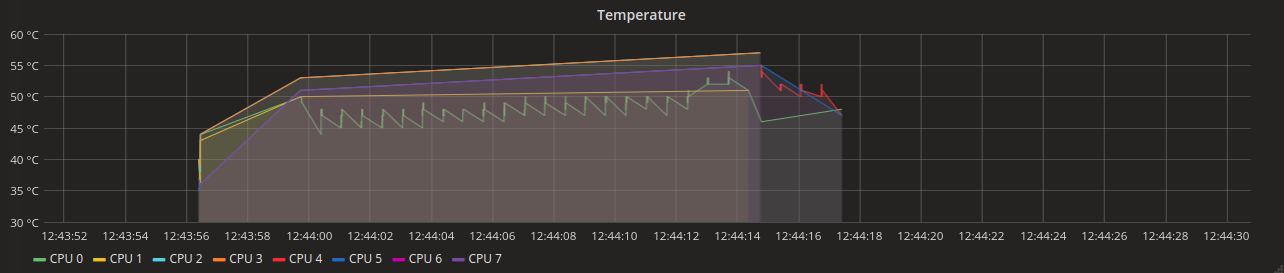##### PMU channel 0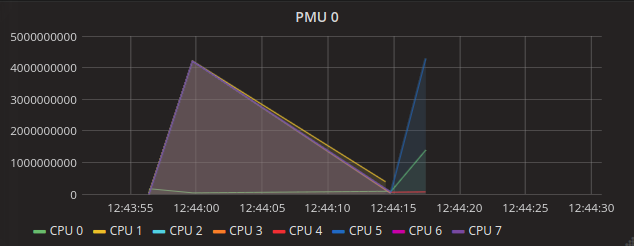##### PMU channel 1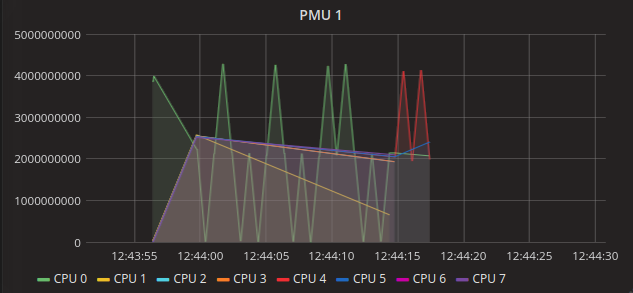##### PMU channel 2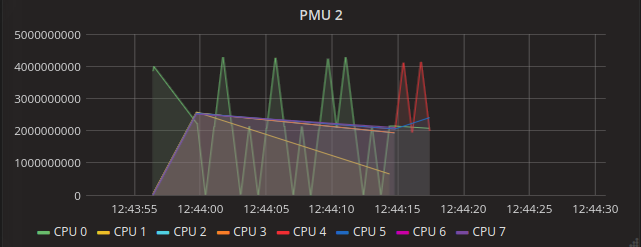##### PMU channel 3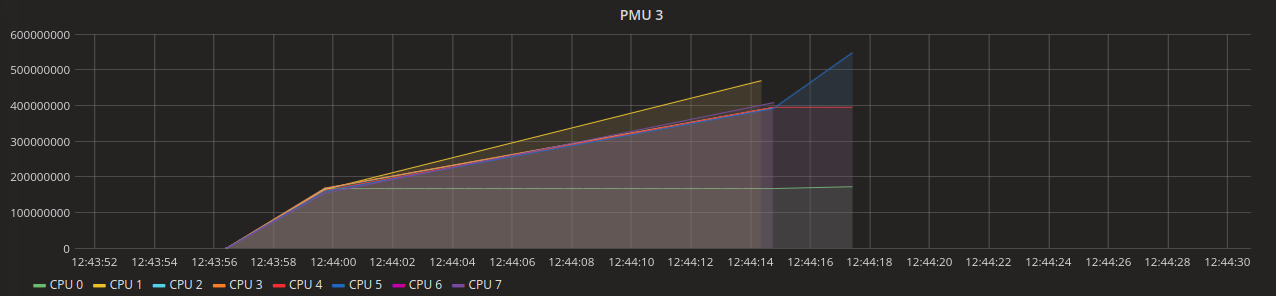##### PMU channel 4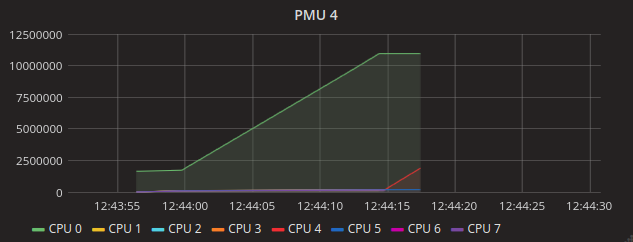##### PMU channel 5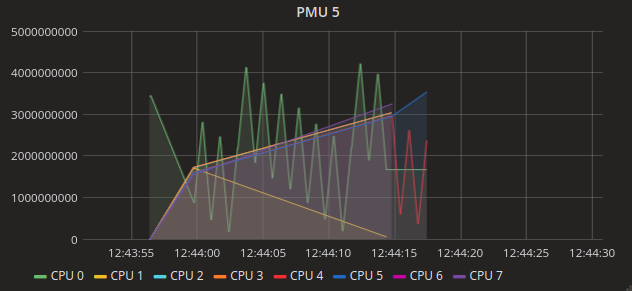##### PMU channel 6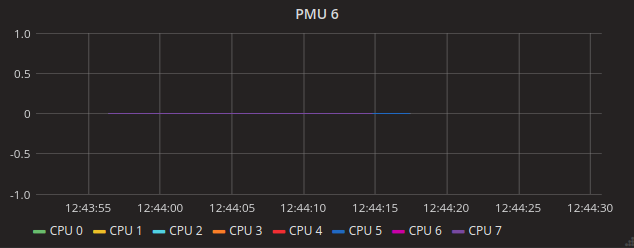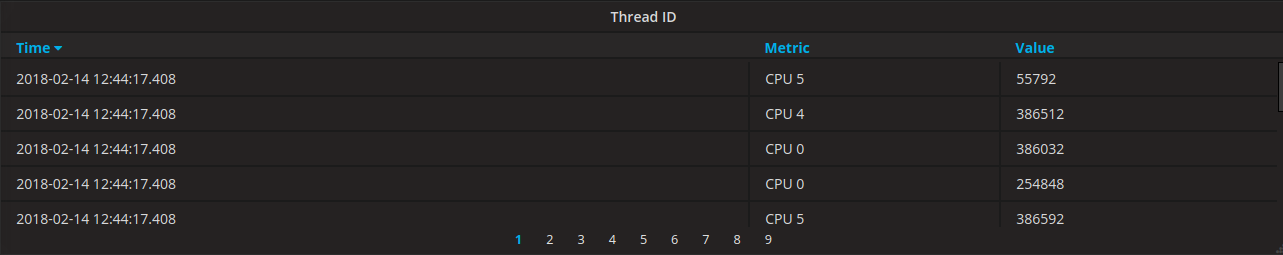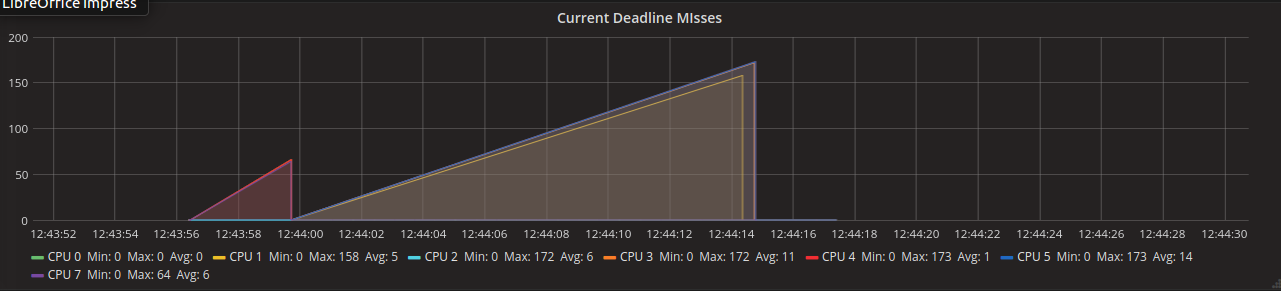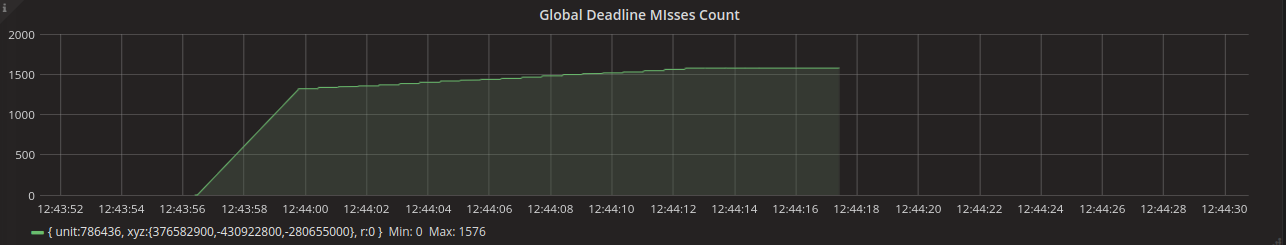## Fifth Version of Monitoring Capture

In this version we have changed many things. First of all, we have changed the way we store the captures and consequently changed the place where we print the data captured. We needed to do this because the old way of printing was generating too many jitters in the execution time (something like 400% of the normal execution time).
So as we can't let this happen any more, we throw away the circular buffer methods of storage and make everything based on a vector allocated outside the system's heap, something like 10MB of the RAM to store the captures of a 120 seconds (approximately) execution.

The complete code is available at Current code.

### Allocating the Buffer

As we say before, we can't allocate a buffer with that great amount of data (~10MB) normally in EPOS. To do that, we need to increase the heap size and use an allocation before the heap be available to the application. It was recommended to increase heap size on the app system's traits file and do the allocation on thread_init.cc. As a initial version, we are allocating 4MB.

#### App System's Traits:

```template<> struct Traits<System>: public Traits<void>
{
static const unsigned int mode = Traits<Build>::MODE;
static const bool multitask = (mode != Traits<Build>::LIBRARY);
static const bool multicore = (Traits<Build>::CPUS > 1) && multithread;
static const bool multiheap = (mode != Traits<Build>::LIBRARY) || Traits<Scratchpad>::enabled;

enum {FOREVER = 0, SECOND = 1, MINUTE = 60, HOUR = 3600, DAY = 86400, WEEK = 604800, MONTH = 2592000, YEAR = 31536000};
static const unsigned long LIFE_SPAN = 1 * HOUR; // in seconds

static const bool reboot = true;

static const unsigned int STACK_SIZE = Traits<Machine>::STACK_SIZE;
__static const unsigned int HEAP_SIZE = (4 * 1024 * 1024) + (Traits<Application>::MAX_THREADS + 1) * Traits<Application>::STACK_SIZE;__
};```

```void Thread::init()
{
...
Criterion::init();

if (Criterion::monitoring) {
if (Machine::cpu_id() == 0){
_end_capture = true;
// Allocating a memeory region (4MB) for store the capture
// For this to work, we increased heap size in 4MB. --> This can handle 64000 captures
_thread_monitor = new Monitoring_Capture(8000, __new (SYSTEM) Moment__);
_end_capture = false;
}
}
...
}```

### Changes in Constructor

The allocation change implies on changing the way we are capturing. So, to do this we need to change the constructor to properly initialize the attributes.
The first thing we do is divide the memory allocated to the CPUs. For this we decide to divide equally to each one of them.
The following remains almost the same, only now we decide to clean up a bit the main of the application, so we print some parameters (series in JSON and the other information's like units and time) in the end of the constructor (print_smart_params() and series()) before the capture's start.

```// int size --> size of each slice of the vector (totalSize/CPUs);
// Moment * init --> The pointer to the initial position of the vector.
Monitoring_Capture(int size, Moment * init) {
_max_size = size;
for (unsigned int i = 0; i < Traits<Build>::CPUS; i++) {
_mem_pos[i] = i * size;
_init_pos[i] = _mem_pos[i];
_over[i] = 0;
}
_mem_moment = init;
_t0 = RTC::seconds_since_epoch() * 1000000;
_t1 = _t0 + (5*60*1000000);
_tsc_base = _t0 - (TSC::time_stamp() * 1000000 / TSC::frequency());
NIC nic;
for (int i = 0; i < 12; i++)
_units[i] = (i+1) << 16 | 4;
_x =  3746654;//3765.829 * 100000;
_y = -4237592;
_z = -293735;
_r = 0;
_errorsmart = 0;
_confidence = 1;
print_smart_params();
series();
}```

#### Calling the constructor (inside Thread::init())

```//Disable captures
_end_capture = true;
// Allocating a memeory region (4MB) for store the capture
// For this to work, we increased heap size in 4MB. --> This can handle 64000 captures
_thread_monitor = new Monitoring_Capture(8000, __new (SYSTEM) Moment__);
_end_capture = false;
//Enable Captures```

### Changes in Monitoring_Capture::capture()

The parameters of the function remains the same. What we change here is where we store the capture. We get the memory position of the CPU that do the capture and then store the new moment in that position. We only let the CPU writes in their respective vector, to do this we verify if the current positions is lower than their respective maximum position, else it will increase a value of overflow (this occur only for us to see if it's needed to increase the vector size).
The vector is divided like this to remove the concurrent write in memory, which would need a lock to not occur overwriting.

```void capture(unsigned int temperature, unsigned int pmu0, unsigned int pmu1, unsigned int pmu2, unsigned int pmu3, unsigned int pmu4, unsigned int pmu5,
if (_mem_pos[cpu_id] < ((cpu_id+1) * _max_size)) {
unsigned int pos = _mem_pos[cpu_id];
_mem_moment[pos]._temperature = temperature;
_mem_moment[pos]._pmu0 = pmu0;
_mem_moment[pos]._pmu1 = pmu1;
_mem_moment[pos]._pmu2 = pmu2;
_mem_moment[pos]._pmu3 = pmu3;
_mem_moment[pos]._pmu4 = pmu4;
_mem_moment[pos]._pmu5 = pmu5;
_mem_moment[pos]._pmu6 = pmu6;
_mem_moment[pos]._time_stamp = _tsc_base + (TSC::time_stamp() * 1000000 / TSC::frequency());
_mem_pos[cpu_id]++;
} else {
_over[cpu_id]++;
}
}```

### Changes in Monitoring_Capture::datas()

It is needed to change the way we print the stored data, and we do this by traversing the vector of the starting position of each CPU until the end of it.
The prints after "<end_capture>" only occur so that we can check some execution information (time and vector overflow).

```void Monitoring_Capture::datas() {
unsigned long long final_time = RTC::seconds_since_epoch() *1000000;
Moment m;
for (unsigned int i = 0; i < Machine::n_cpus(); i++) {
for (unsigned int j = _init_pos[i]; j < _mem_pos[i]; j++) {
m = _mem_moment[j];
unsigned long long value[] = {m._temperature, m._pmu0, m._pmu1, m._pmu2, m._pmu3, m._pmu4, m._pmu5, m._pmu6,
cout << "<";
for (int i = 0; i < 13; i++) {
cout << value[i] << ",";
}
cout << value << ">" << endl;
}
}
cout << "<end_capture>" << endl;
for (unsigned int i = 0; i < Machine::n_cpus(); i++) {
cout << "cap  CPU" << i << ": " << _mem_pos[i] - _init_pos[i] << endl;
cout << "over CPU" << i << ": " << _over[i] << endl;
}
cout << "final time | Elapsed time: " << final_time << " | " << (final_time - _t0)/1000000 << endl;
while(1) cout<<"simulation ended"<<endl;
}```

### Calling Monitoring_Capture::datas()

The method is called in the end of the application execution, before Thread::idle() calls the reboot and halt.

```int Thread::idle()
{
while(_thread_count > Machine::n_cpus()) { // someone else besides idles
db<Thread>(TRC) << "Thread::idle(CPU=" << Machine::cpu_id() << ",this=" << running() << ")" << endl;
CPU::int_enable();
CPU::halt();
if(_scheduler.schedulables() > 0) // A thread might have been woken up by another CPU
yield();
}

CPU::int_disable();
if(Machine::cpu_id() == 0) {
if (Criterion::monitoring)

if(reboot) {
db<Thread>(WRN) << "Rebooting the machine ..." << endl;
Machine::reboot();
} else
db<Thread>(WRN) << "Halting the machine ..." << endl;
}
CPU::halt();

return 0;
}```

## Sending smartdata in a faster way

As we generate a very large amount of smartdatas per execution, the normal way to send it, as a JSON, takes too much time to run. With the help of LISHA's IoT developers we improved the system to send the data in a faster way using a certificate other than a login and password, and sending it using the Binary API.

### To send we used a python script based on the eposiotgw, available on 'epos/tools/eposiotgw/'.

The idea was to read the log and generate the byte sequence, so we could store it in a file that python script could read and send. The main purpose behind all those operations was to save time and do not use the computer (used to run the test) resources.
During the configuration of the python script, we got into some problems using python to read a file containing binaries. The code used to read (both ones, opening in read mode with or without the binary flag) brought the 'string' with differences and strange characters, i.e., the string read was different of the string in the file.
As a solution to the problem, we change the c++ code that generates the binary file to write a sequence of integers (each one representing one byte), so the python file opens it, read and convert to binary the read integers and then send it using the binary API.

### Code:

##### Python script:
```#!/usr/bin/env python3

# To get an unencrypted PEM (without passphrase):
# openssl rsa -in certificate.pem -out certificate_unencrypted.pem

import os
import sys
import time
#import serial
import argparse
import requests
import json

parser = argparse.ArgumentParser(description='EPOS Serial->IoT Gateway')

parser.add_argument('-d','--dev', help='EPOSMote III device descriptor file', default='/dev/ttyACM0')

args = vars(parser.parse_args())
DEV = args['dev']
TIMEOUT = int(args['timeout'])
URL = args['url']
ATTACH_URL = args['attach_url']
MY_CERTIFICATE = [args['certificate']+'.pem', args['certificate']+'.key']
DOMAIN = args['domain']
JSON = args['json']

with open('strings.bin') as f:
array = [int(x) for x in f.read().split(',')]

inp = bytes(array)
session = requests.Session()
session.headers = {'Connection': 'close', 'Content-type' : 'application/octet-stream', 'Content-length' : str(51)}
session.cert = MY_CERTIFICATE

try:
#print(inp)
response = session.post(URL, inp)
#print("[", str(response.status_code), "] (", len(d), ") ", d, sep='')
print("SEND", str(response.status_code), str(response.text))
except Exception as e:
#print("Exception caught:", e, file=sys.stderr)
print("Exception caught:", e)

#print d```

##### c++ functions used to convert the DB_Records to binaries
```#include <stdio.h>
#include <iostream>
#include <stdlib.h>
#include <fstream>
#include "ListaEnc.hpp"
#include <unistd.h>
#include <string.h>
#include <bitset>
#include <math.h>
...
std::string reverterBytes(std::string src) {
std::string dst = "";
std::string aux[src.size()/8];

for (int i = 0; i < src.size(); i+=8) {
aux[i/8] = "";
aux[i/8] += src[i];
aux[i/8] += src[i+1];
aux[i/8] += src[i+2];
aux[i/8] += src[i+3];
aux[i/8] += src[i+4];
aux[i/8] += src[i+5];
aux[i/8] += src[i+6];
aux[i/8] += src[i+7];
}

for (int i = (src.size()/8)-1; i >= 0 ; i--) {
dst += aux[i];
}

return dst;
}

struct DB_Series {
unsigned char version;
unsigned long unit;
long x;
long y;
long z;
unsigned long r;
unsigned long long t0;
unsigned long long t1;
};

struct DB_Record {
unsigned char version;
unsigned long unit;
double value;
unsigned char error;
unsigned char confidence;
long x;
long y;
long z;
unsigned long long t;
unsigned char mac;
};
...
void send_binary_records(/*std::string to_send*/ DB_Record reg) {
std::string r("");
std::string a[] = {"A", "B", "C", "D", "E", "F"};
std::string aux = "";
int v = 0;
int acum = 0;
if (binaries < 108) {
// converting DB_Record.value to bytes
char *bytes = reinterpret_cast<char *>(&reg.value);

// the following code is used to convert to a complete binary
// (we use this because only converting to char * ignore the left '0's)
// and ignoring it will cause to the data sended via http request to be out of format
r += reverterBytes(std::bitset<1*8>(reg.version).to_string());
r += reverterBytes(std::bitset<4*8>(reg.unit).to_string());
for(int i = 0; i < 8; i++) {
r += reverterBytes(std::bitset<1*8>(bytes[i]).to_string());
}
r += reverterBytes(std::bitset<1*8>(reg.error).to_string());
r += reverterBytes(std::bitset<1*8>(reg.confidence).to_string());
r += reverterBytes(std::bitset<4*8>(reg.x).to_string());
r += reverterBytes(std::bitset<4*8>(reg.y).to_string());
r += reverterBytes(std::bitset<4*8>(reg.z).to_string());
r += reverterBytes(std::bitset<8*8>(reg.t).to_string());
r += reverterBytes(std::bitset<16*8>(0).to_string());

// as we get the binary above, we still need to convert it to a byte form (\xvv)
// where \x correspons to hexa format and v is a hexa number (0...F).
// The problem is that we can't write it direct as a hexa number, because python can't read it perfectly
// So, we write it as an integer that represent that byte (0...255) and after convert it to a byte.
for (int i = 0; i < r.size(); i+=8) {
aux += r[i];
aux += r[i+1];
aux += r[i+2];
aux += r[i+3];
aux += r[i+4];
aux += r[i+5];
aux += r[i+6];
aux += r[i+7];

for (int j = 0; j < 8; j++) {
v = aux[j]-48;
acum += v * pow(2, 8-(j+1));
}

// for (int j = 4; j < 8; j++) {
// 	v = aux[j]-48;
// 	acum += v * pow(2, 8-(j+1));
// }

binary_string += std::to_string(acum);
if (i < r.size() -8)
binary_string += ",";
acum = 0;
aux = "";
}
binary_string+=",";
} else {
char *bytes = reinterpret_cast<char *>(&reg.value);
r += reverterBytes(std::bitset<1*8>(reg.version).to_string());
r += reverterBytes(std::bitset<4*8>(reg.unit).to_string());
for(int i = 0; i < 8; i++) {
r += reverterBytes(std::bitset<1*8>(bytes[i]).to_string());
}
r += reverterBytes(std::bitset<1*8>(reg.error).to_string());
r += reverterBytes(std::bitset<1*8>(reg.confidence).to_string());
r += reverterBytes(std::bitset<4*8>(reg.x).to_string());
r += reverterBytes(std::bitset<4*8>(reg.y).to_string());
r += reverterBytes(std::bitset<4*8>(reg.z).to_string());
r += reverterBytes(std::bitset<8*8>(reg.t).to_string());
r += reverterBytes(std::bitset<16*8>(0).to_string());

for (int i = 0; i < r.size(); i+=8) {
aux += r[i];
aux += r[i+1];
aux += r[i+2];
aux += r[i+3];
aux += r[i+4];
aux += r[i+5];
aux += r[i+6];
aux += r[i+7];

for (int j = 0; j < 4; j++) {
v = aux[j]-48;
acum += v * pow(2, 8-(j+1));
}

for (int j = 4; j < 8; j++) {
v = aux[j]-48;
acum += v * pow(2, 8-(j+1));
}

binary_string += std::to_string(acum);
if (i < r.size() -8)
binary_string += ",";
acum = 0;
aux = "";
}
std::ofstream out;
out.open("strings.bin", std::ios::out | std::ios::binary);
out<<binary_string;
out.close();
binary_string="";
system("./smartdata_sender -c client-8-A7B64D415BD3E982");
binaries=0;
//system("rm -f strings.bin");*/
}
binaries++;
}
...```

The complete code is available at sending_with_binary_API

### Binary API config

After some tests, we decided to send 108 DB_Records per script execution. Turning the send time from almost 3 hours to less then 30 minutes (sending about 5000 captures, or 60000 DB_Records). It is possible to send more DB_Records, but with lost of performance (we tried 120 and lost about 3 minutes more).

### Batch API

The idea behind Batch API is to send more data together, decreasing the overhead of the HTTP protocol using a special type of insertion on the Data Base.

### Code Changes

• sender.cc
• Modify in the current methods for the new way the deadline per thread series are arranged.
• New Method to convert a series to binary.
• New method for empty the file, now we need to insert the series at the beginning. The Batch insert is organized as {db_series, db_record1, .., db_recordN}.
• Modify the sending method to send only when reach a cluster of 1200 DB_record or the data file has ended.
• smart_data_sender
• The only thing that we need to change here was the Post URL, that now is defined as "https://iot.lisha.ufsc.br/api/put_batch.php"

### Series Description

Every series with the same t0 and t1 correspond to the same execution.
Digital smart_data: Type << 16 | Size; Our size will be always 4 → 4 bytes → unsigned int
Types From 1...7 (except 4) → One per core, you can easily differs one from another by the locations _x + (cpu_id * 10).
Type 4: To differs one from another by the location of the data, so you just need to sum _x+(80+thread_number), where thread_number is defined as creation order.

 TYPE VALUE DESCRIPTION o (0+1) << 16 OR 4 = 65540 Global Deadline Miss. Only one to all cores. 1 (1+1) << 16 OR 4 = 131076 Temperature. 2 (2+1) << 16 OR 4 = 196612 Thread_id. 3 (3+1) << 16 OR 4 = 262148 Thread_Priority. 4 (4+1) << 16 OR 4 = 327684 Deadline per thread. 5 (5+1) << 16 OR 4 = 393220 PMU Fixed channel_0. 6 (6+1) << 16 OR 4 = 458756 PMU Fixed channel_1. 7 (7+1) << 16 OR 4 = 524292 PMU Fixed channel_2. N ((PMU: :_channel_N+8)+1) << 16 OR 4 PMU configurable channels.

Where PMU: :_channel_N is the value of the position of the event into PMU::Event enum defined on pmu.h (common). The configurable events from Intel Sandy_Bridge available on EPOS (206 in total) starts at position 12.

### Final Version and Description

The Final Version of the code can be found at code_link.

#### List of files change (from trunk code)

• monitoring_capture.h (include/architecture/ia32)
• monitoring_capture_send.cc (src/architecture/ia32)
• scheduler.h (include)
• cpu.h (include/architecture/ia32)
• alarm.cc (src/component)
• semaphore.cc (src/component)
• types.h (include/system)
• rtc.h (include)
• pmu.h (include)
• pmu.cc (src/architecture/ia32)
• pmu.h (include/architecture/ia32)
• test_time(.cc, _traits.h) (app) → special attention on traits file.
• semaphore.cc (src/component)

#### Description and tips

• The capture logic is defined on monitoring_capture.h.
• The print logic is defined at monitoring_capture_send.cc.
• In thread.h we define the variable used during the execution.
• The number of captures per CPU is defined in thread_init, but if you want to increase, take a look at heap size at traits (each 8000 captures per CPU, add 1 at the first multiplier of the size calculum).
• The capture is done every time Thread::dispatch() runs.
• The number of deadlines is calculated in semaphore v, and the local deadlines (per thread) is calculated on dispatch (using values from periodic_thread).
• Temperature is read from CPU, a method that is available only in the new versions of the code.
• To avoid some jitter on the alarm, the original if was replaced by a while.
• The events on the pmu.h were ordered as the enum on the PMU::Sandy_Bridge (see pmu.h in include/architecture/ia32).
• There’s a tip: if you add any event on include/pmu.h::Event, remember to change the code at src/architecture/ia32/pmu.cc, and, if you want it to keep the easy configure of the events that will be captured, add at the final of the enum, right before EVENTS (it’s an integer that has the value equals to the size of the enum).
• To enable the capture you just need to use one of the scheduling criterias we have developed (MCEDF, MPEDF or MGEDF) - To disable, change it.
• If you want to use the captures with other scheduling criteria you just need to enable the flag monitoring and configure the PMU (In most of the cases you just need to copy and paste what we have done in the init() method of MCDEF, MPEDF or MGEDF).
• If you create a new Scheduling Criteria don’t forget to define it on types.h and what is the queue that it uses (end of scheduler.h file).
• The rtc.h code has an error on the seconds_since_epoch() method, take care if you are going to replace it.
• The test is controlled to avoid unnecessary captures using a variable named _end_capture (on Thread class), if you want to change this logic, it is possible, change the if on dispatch to do the same check used on idle method (Thread class, both), but you will do a few more captures (the main thread still running until the idle start the print code execution).
• All the data captured is printed after the execution, though it spends a lot of memory, it is the best way to avoid I/O jitter.
• The total execution time is printed at the end of the log. This time counts the series printing time. To get the actual execution time, see the value printed before <begin_tseries>, on the log, this is the time spent to execute the main function.
• The values printed between <end_series> and time are tuples containing each thread period and number of iterations it should execute to run till the end of the execution(executions).
• All the values on the log (except time, iteration tuples, DATE and anything that appears after the <end_capture> - in general, number of captures per CPU) are used as parameters to the sending code, so, do not change the printing structure.
• The over capture counting is disabled, to avoid jitters, if you need it, uncomment the code.

If you have any doubt, almost all of the steps we have done to achieve this code is described on the previous topics.

#### PMU Events

To configured events to be captured you must go to include/pmu.h (EPOS 2.1) and configure channel_3 parameter to the number of the event:

• Ex.: static const unsigned int _channel_3 = 214;

#### Send code files

• sender.cc
• smartdata_sender (python configured to run with python 3 - use ./smartdata_sender)
• series.py
• the certificates
• series.log (where you should put the log)
• strings.json (where the code puts the series to send - python reads it)
• strings.bin (where the code puts the bin to send the smartdatas - python reads it)
• list_element.hpp

The current version of this sender is configured to send using batch API. If it does not work, check the certificates. If it still does not work, check the current version of the API with the supporters.

#### Compiling Scripts

• batch
• batch_comp.sh <app_name>
• simple
• comp.sh <app_name>

Both are configured to send image using the configuration of the ssh as entropy_proxy, change it to use other machine.
Executing sh:
if you got some kind of execution permission fault, try: sudo chmod +x <name>.sh
./<name>.sh <app_name>

#### Executing and sending Script

• batch_execution.sh
• Copies a image from the specified (inside the code) PATH to the machine boot folder as epos.img;
• Makes a system call to execute the python (PCcontrol.py) to turn on the machine, with the sending flag up;
• Repeat the previous steps until it consumes all the images on the specified PATH;

• PCControl.py
• Flags
```usage: PCControl.py [-h] [-c COMMAND] [-t COMMENT] [-a AUTHOR] [-n NAME] [-mail E_MAILS] [-s AUTO_SEND] [-to TIME_OUT]

optional arguments:
-h, --help            show this help message and exit
-c COMMAND, --command COMMAND
1 -> On | 0 -> Off
-t COMMENT, --comment COMMENT
add a commenet in the beginning of the log
-a AUTHOR, --author AUTHOR
put your name in the log
-n NAME, --name NAME  rename the log
-to TIME_OUT, --time_out TIME_OUT
max running_time in seconds
-mail E_MAILS, --e_mails E_MAILS
e-mails to advice when simulation is ended
-s AUTO_SEND, --auto_send AUTO_SEND
send to smart-data after capture```

• This is the main script, here we can turn ON/OFF the PC that boot and runs the epos application.
• This code also reads the serial port and fills the log file (name-author-date.log).
• With the optional flag “-mail”, you can notify the email list (‘email1,email2,...,emailN’) provided when the execution has ended.
• With the optional “-s” flag, you run sending code when the other things have ended their execution.

### Next to do!

1. Generate a great amount of data to apply an ANN to use Machine Learning

## Bibliography

1. EPOS DOCUMENTATION: http://epos.lisha.ufsc.br/EPOS+Documentation;
2. PROJECT FIRST TRIMESTER: https://lisha.ufsc.br/article560;
3. PROJECT SECOND TRIMESTER: https://lisha.ufsc.br/article561;